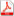Issue 4, 2019 Vol. 11

# All issues

Designing a zero on a linear manifold, a polyhedron, and a vertex of a polyhedron. Newton methods of minimizationpdf (477K)  / List of references

We consider the approaches to the construction of methods for solving four-dimensional programming problems for calculating directions for multiple minimizations of smooth functions on a set of a given set of linear equalities. The approach consists of two stages.

At the first stage, the problem of quadratic programming is transformed by a numerically stable direct multiplicative algorithm into an equivalent problem of designing the origin of coordinates on a linear manifold, which defines a new mathematical formulation of the dual quadratic problem. For this, a numerically stable direct multiplicative method for solving systems of linear equations is proposed, taking into account the sparsity of matrices presented in packaged form. The advantage of this approach is to calculate the modified Cholesky factors to construct a substantially positive definite matrix of the system of equations and its solution in the framework of one procedure. And also in the possibility of minimizing the filling of the main rows of multipliers without losing the accuracy of the results, and no changes are made in the position of the next processed row of the matrix, which allows the use of static data storage formats.

At the second stage, the necessary and sufficient optimality conditions in the form of Kuhn–Tucker determine the calculation of the direction of descent — the solution of the dual quadratic problem is reduced to solving a system of linear equations with symmetric positive definite matrix for calculating of Lagrange's coefficients multipliers and to substituting the solution into the formula for calculating the direction of descent.

It is proved that the proposed approach to the calculation of the direction of descent by numerically stable direct multiplicative methods at one iteration requires a cubic law less computation than one iteration compared to the well-known dual method of Gill and Murray. Besides, the proposed method allows the organization of the computational process from any starting point that the user chooses as the initial approximation of the solution.

Variants of the problem of designing the origin of coordinates on a linear manifold, a convex polyhedron and a vertex of a convex polyhedron are presented. Also the relationship and implementation of methods for solving these problems are described.

Keywords: Newton methods, quadratic programming, dual quadratic problem, sparse matrices, Cholesky factorization, direct multiplicative algorithm, numerical stability, zero design problem, linear manifold, vertex of a polyhedron
Citation in English: Sviridenko A.B. Designing a zero on a linear manifold, a polyhedron, and a vertex of a polyhedron. Newton methods of minimization // Computer Research and Modeling, 2019, vol. 11, no. 4, pp. 563-591
Citation in English: Sviridenko A.B. Designing a zero on a linear manifold, a polyhedron, and a vertex of a polyhedron. Newton methods of minimization // Computer Research and Modeling, 2019, vol. 11, no. 4, pp. 563-591
DOI: 10.20537/2076-7633-2019-11-4-563-591
Views (last year): 6.

Full-text version of the journal is also available on the web site of the scientific electronic library eLIBRARY.RU

The journal is included in the Russian Science Citation Index

The journal is included in the List of Russian peer-reviewed journals publishing the main research results of PhD and doctoral dissertations.

International Interdisciplinary Conference "Mathematics. Computing. Education"

The journal is included in the RSCI

Indexed in Scopus